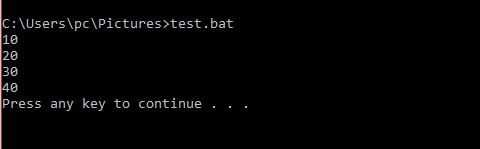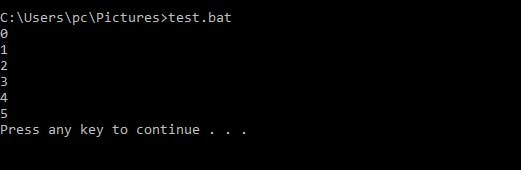# batch file for loopLike other programing language batch script support the looping. In this article, I will explain the batch file for loop and describe some example to how we can use the batch file for loop in our program.

### Batch File For Loop Syntax,

#### Parameters for the batch script for loop

%%VarName => declaration of the variable for the “for loop” executed once.

Note: for the command prompt you can use %VarName.

List => list is the values for which for loop will be executed.

iteration_Code =>  Code block which is executed for each iteration.

You can see this article, Batch file commands.

### Batch file for loop example

In the above program, I have created a list which contains the elements 10, 20,30 and 40. So, for every element of the list, the for loop will be executed and print the corresponding value.### Batch script for loop for the range values (FOR /L)

In the batch file, we can also execute the statements according to the given range.

Syntax,

/L => /L signifies that for loop is used for iterating through a range of values.

Expression1: It is an initial value ( lower limit )for the loop.

Expression2: It is the value which will add in the initial value after each iteration.

Expression3: It is the last number for the loop.

Note: If three expressions are 0, then the body will execute indefinitely.

Let see an example, where I am echoing value from 0 to 5

When you will run the above batch file, you will get below output.